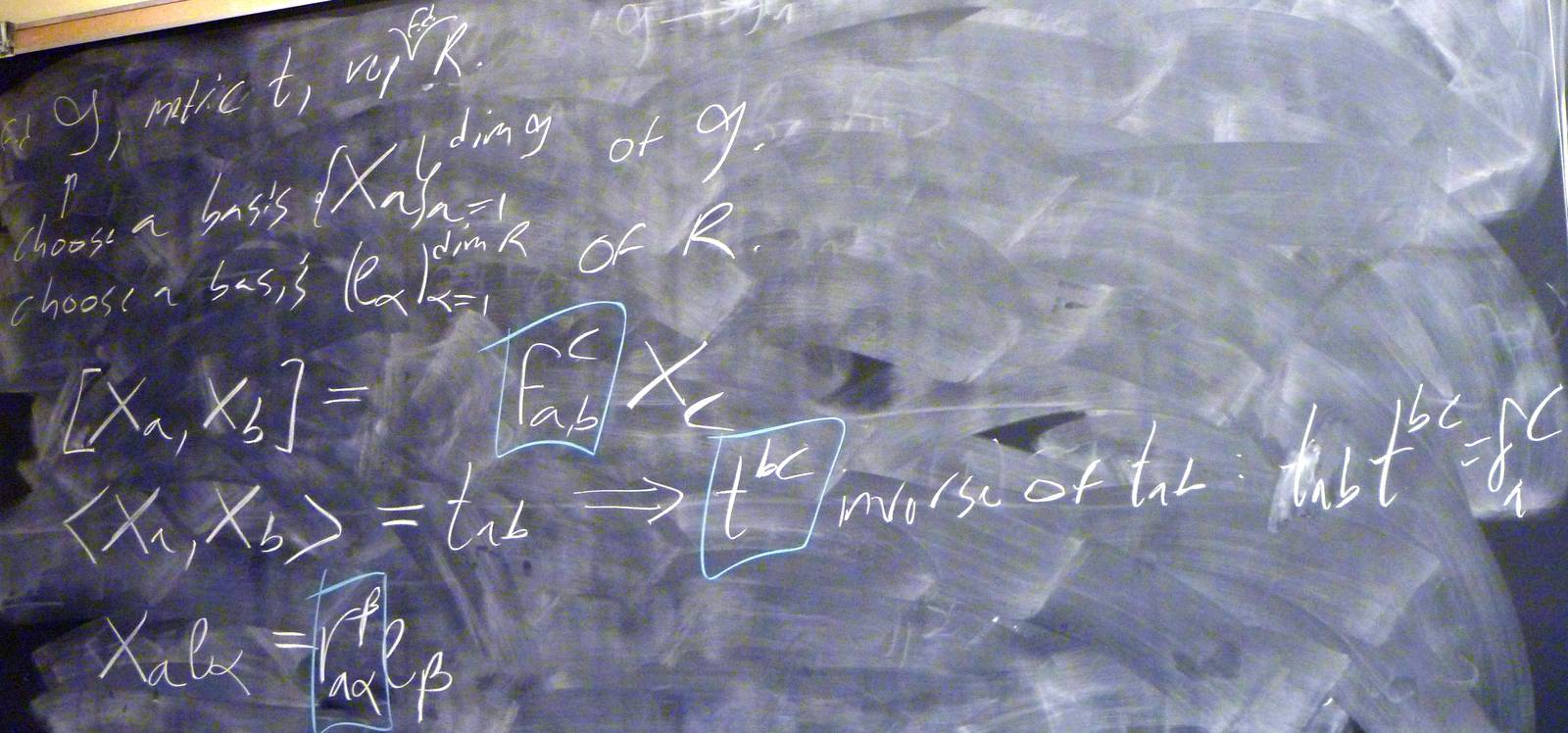© | Dror Bar-Natan: Classes: 2013-14: AKT: < >

# AKT-140224 Video

 width: 400 720 orig/AKT-140224.MOD For now, this video can only be viewed with web browsers that support

Notes on AKT-140224:    [edit, refresh]

A review of Lie algebras.

# Week of... Notes and Links
1 Jan 6 About This Class (PDF).
: Course introduction, knots and Reidemeister moves, knot colourings.
Tricolourability without Diagrams
: The Gauss linking number combinatorially and as an integral.
: The Schroedinger equation and path integrals.
Friday Introduction (the quantum pendulum)
2 Jan 13 Homework Assignment 1.
: The Kauffman bracket and the Jones polynomial.
: Self-linking using swaddling.
: Euler-Lagrange problems, Gaussian integration, volumes of spheres.
3 Jan 20 Homework Assignment 2.
: The definition of finite-type and some examples.
: The self-linking number and framings.
: Integrating a polynomial times a Gaussian.
Class Photo.
4 Jan 27 Homework Assignment 3.
: Chord diagrams and weight systems.
: Swaddling maps and framings, general configuration space integrals.
: Some analysis of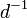$d^{-1}$.
5 Feb 3 Homework Assignment 4.
: 4T, the Fundamental Theorem and universal finite type invariants.
The Fulton-MacPherson Compactification (PDF).
: The Fulton-MacPherson Compactification, Part I.
: More on pushforwards,$d^{-1}$, and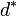$d^\ast$.
6 Feb 10 Homework Assignment 5.
: The bracket-rise theorem and the invariance principle.
: The Fulton-MacPherson Compactification, Part II.
: Gauge fixing, the beginning of Feynman diagrams.
R Feb 17 Reading Week.
7 Feb 24 : A review of Lie algebras.
: Graph cohomology and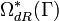$\Omega_{dR}^\ast(\Gamma)$.
: More on Feynman diagrams, beginning of gauge theory.
From Gaussian Integration to Feynman Diagrams (PDF).
8 Mar 3 Homework Assignment 6 (PDF)
: Lie algebraic weight systems.
: Graph cohomology and the construction of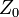$Z_0$.
Graph Cohomology and Configuration Space Integrals (PDF)
: Gauge invariance, Chern-Simons, holonomies.
Mar 9 is the last day to drop this class.
9 Mar 10 Homework Assignment 7 (PDF)
: The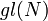$gl(N)$ weight system.
: The universal property, hidden faces.
: Insolubility of the quintic, naive expectations for CS perturbation theory.
10 Mar 17 Homework Assignment 8 (PDF)
: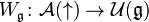$W_{\mathfrak g}\colon{\mathcal A}(\uparrow)\to{\mathcal U}({\mathfrak g})$ and PBW.
: The anomaly.
: Faddeev-Popov, part I.
Gaussian Integration, Determinants, Feynman Diagrams (PDF).
11 Mar 24 Homework Assignment 9 (PDF)
: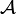${\mathcal A}$ is a bi-algebra.
: Understanding and fixing the anomaly.
Friday: class cancelled.
12 Mar 31 Monday, Wednesday: class cancelled.
: A Monday class: back to expansions.
E Apr 7 : A Friday class on what we mostly didn't have time to do.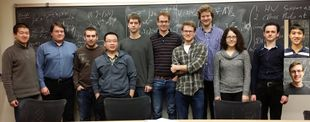Add your name / see who's in!
Dror's Notebookrefresh
panel
Managed by dbnvp: Tip: blackboard shots are taken when the discussion of their content has just ended. To see the beginning of the discussion on a certain blackboard, roll the video to the time of the preceeding blackboard.0:22:08  We will check that the prospective Lie algebra $g = F^2$ satisfies the Jacobi identity $[x,[y,z]] + [y,[z,x]] + [z,[x,y]] = 0$, and thus is indeed a Lie algebra. In contrast with the notation given in lecture, let $\alpha$ and $\beta$ be the basis elements of $F^2$ over $F$, so that $F^2 = \{a\alpha + b\beta: a, b \in F\}$. Now, let $x = a_1\alpha + a_2\beta$, $y = b_1\alpha + b_2\beta$, and $z = c_1\alpha + c_2\beta$ be arbitrary elements in $F^2$.

Using bilinearity,

$[y,z] = b_1c_1[\alpha,\alpha] + b_1c_2[\alpha, \beta] + b_2c_1[\beta,\alpha] + b_2c_2[\beta,\beta]$

Since $[\alpha, \alpha] = [\beta,\beta] = 0$, $[\alpha, \beta] = \alpha$, and $[\beta,\alpha] = -\alpha$, this evaluates to $$[y,z] = (b_1c_2 - b_2c_1)\alpha$$ Similar calculations yield $$[z,x] = (c_1a_2 - c_2a_1)\alpha$$ and $$[x,y] = (a_1b_2 - a_2b_1)\alpha$$ Thus, $$[x,[y,z]] + [y,[z,x]] + [z,[x,y]] = (a_2(b_1c_2 - b_2c_1) + b_2(c_1a_2-c_2a_1) + c_2(a_1b_2+a_2b_1))[\beta, \alpha]$$ $$= -\alpha(a_2b_1c_2 - a_2b_2c_1 + a_2b_2c_1 - a_1b_2c_2 + a_1b_2c_2 - a_2b_1c_2) = 0$$

As a result, the Jacobi identity holds.0:27:53  Lie algebra of dimensions 1 and 2

1. one-dimensional Lie algebras are unique up to isomorphism. For if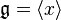$\mathfrak{g} = \langle x \rangle$ is a one dimensional Lie algebra, then since the bracket is antisymmetric, we have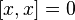$[x, x] = 0$. Thus the bracket is zero and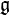$\mathfrak{g}$ is unique up to isomorphism.

2.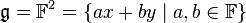$\mathfrak{g} = \mathbb{F}^2 = \{ax + by \;|\; a, b \in \mathbb{F}\}$.$\mathfrak{g}$ is a two-dimensional Lie algebra. There are only two of such up to isomorphism, that is, the one with the bracket equal to zero and the other with bracket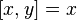$[x, y] = x$.

0:27:53 [add] Lie algebras and representations.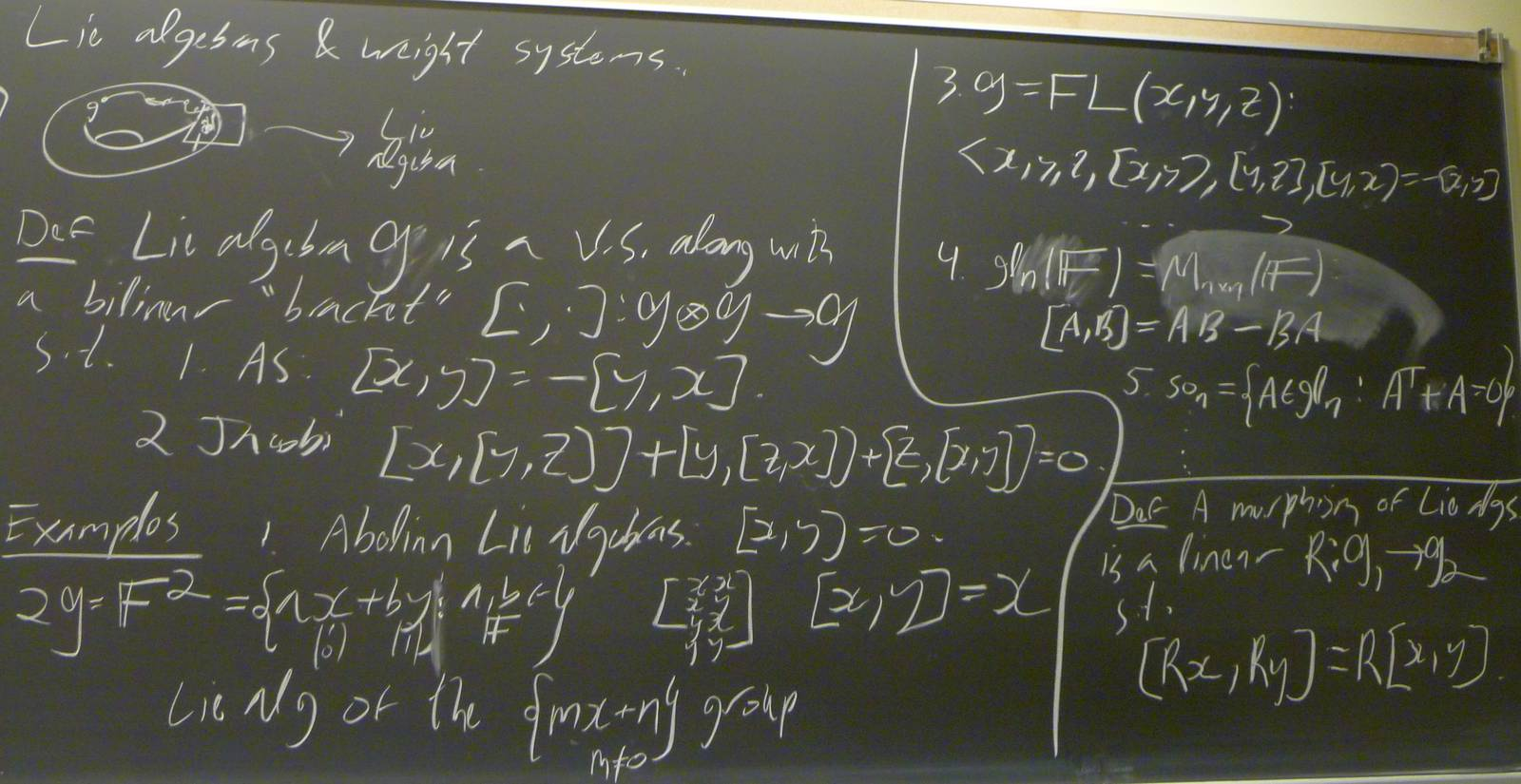0:37:01 [add] Constructing weight systems from Lie algebras.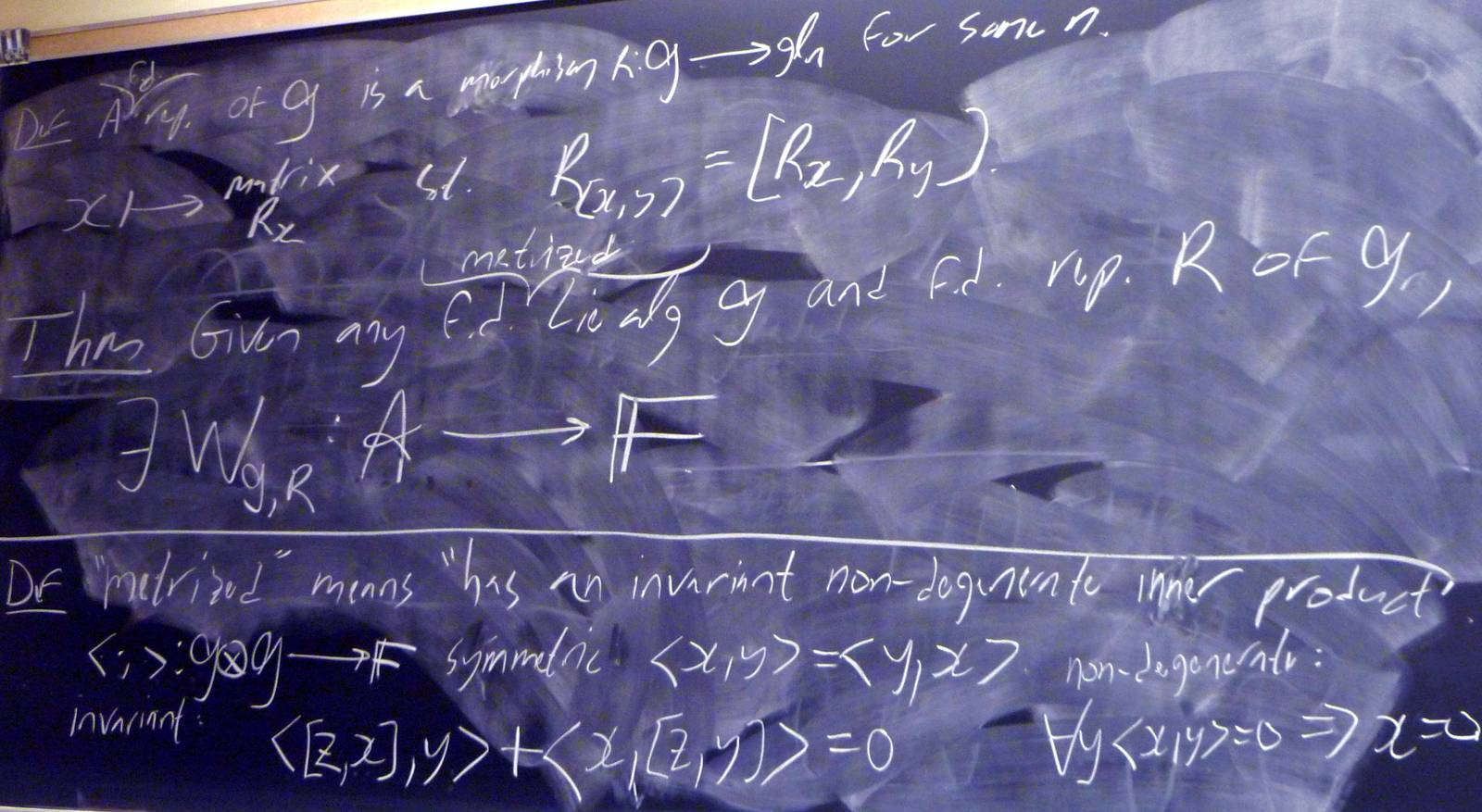0:46:32 [add] Metrized Lie algebras.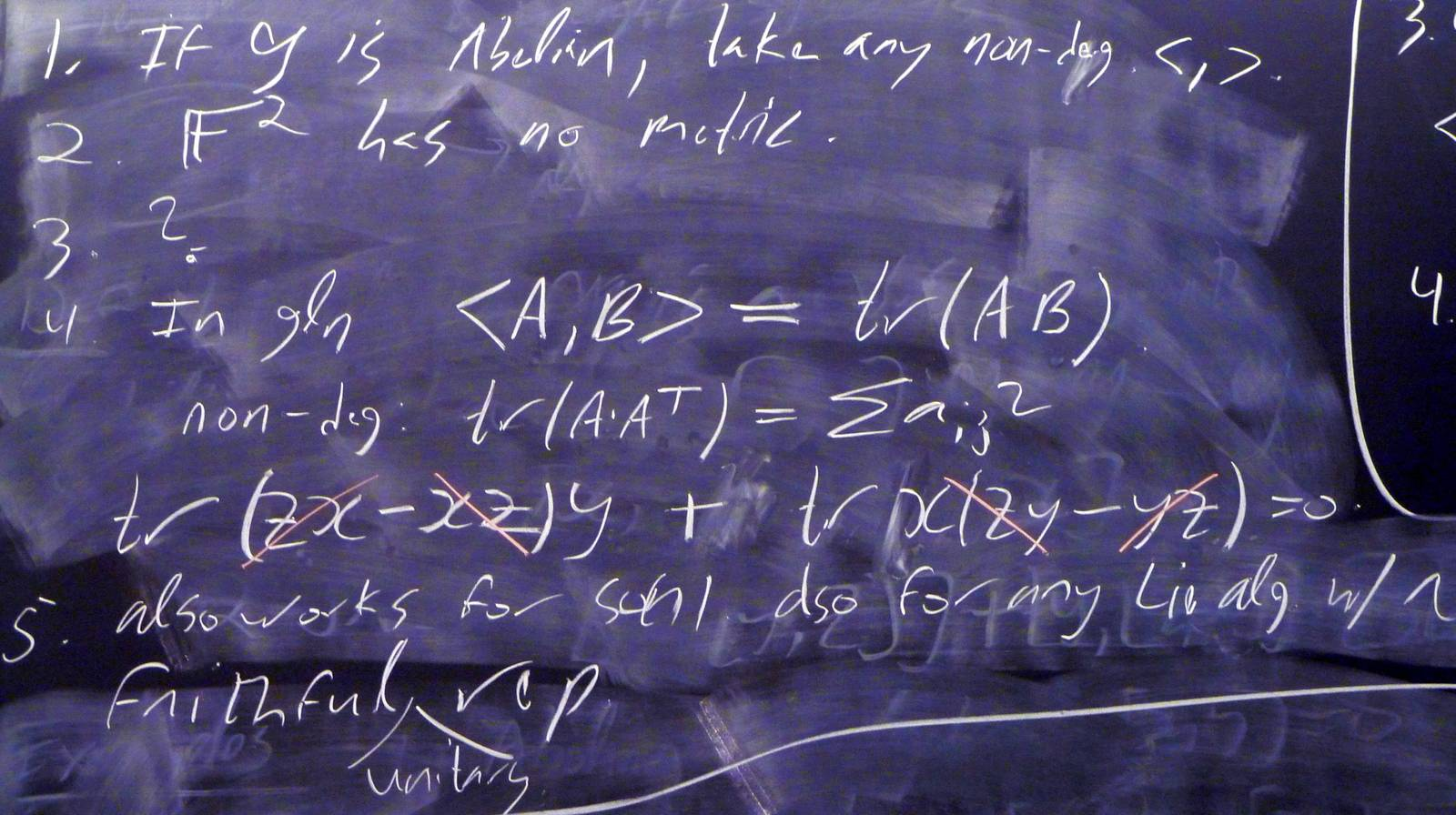0:48:29  The last few minutes of this video were lost for an unclear reason.
0:51:46 [add] The structure constants.